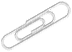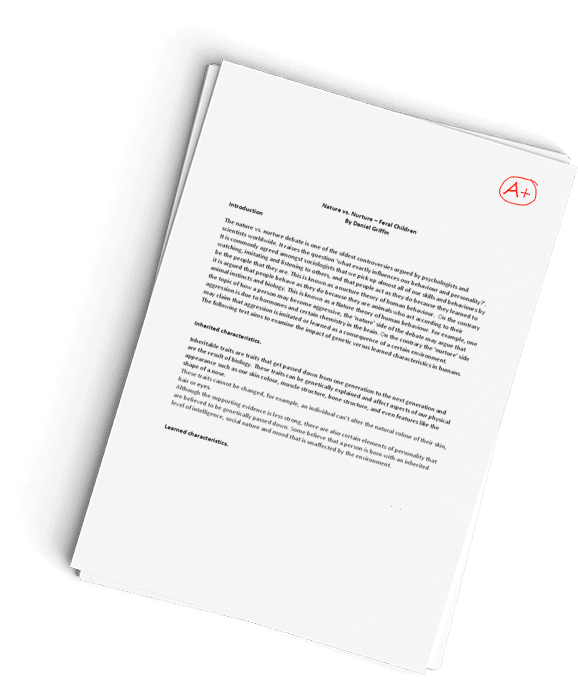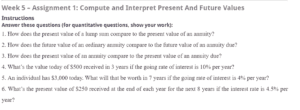Get a timely done, PLAGIARISM-FREE paper
from our highly-qualified writers!## Compute and Interpret Present And Future Values

Sample Answer## Compute and Interpret Present And Future Values

### How does the present value of a lump sum compare to the present value of an annuity?

The present value of a lump sum refers to the total amount that will be required today at a specific discount in order to get a certain amount in the future. In a situation where one wants to conceptualize the required value as one amount, it is referred to as a lump sum. For instance, one may want to know the amount required today to earn a specific future amount at a specified interest rate. In a situation where the amount will be raised annually, the amount is referred to as the present value of an annuity. The difference between the two is the way discount is factored for. In the case of lumpsum, the discount is calculated in wholesome, with the annual discount rate acting as the power in the discount radical. In the present value of an annuity, the power is negative of the annual discount rate. This means that the power is 1 divided by the annual discount rate (Arnold,2013).

### How does the future value of an ordinary annuity compare to the future value of an annuity due?

The future value of an ordinary annuity, according to Arnold (2013), refers to the amount that we expect in the future after investing a certain amount at present. Basically, this means that if you have a specific amount of money and decide to invest it at a certain interest rate, the amount will increase in the future. The amount that accumulates at a specified future date is referred to as the future value of an ordinary annuity. In contrast, if the annuity is already due, it translates as the amount that has already been determined and is due for use. The only issue here is that the future value has not been determined.

### How does the present value of an annuity compare to the present value of an annuity due?

The difference between the PV of an annuity and the PV of an annuity is that the PV of an annuity represents the present value of a future lump sum that is discounted using an annual rate. In essence, the goal here is to know the money that should be held at present to hold a specified amount in the future. The present value of an annuity due is a different concept. As Arnold (2013) points out, the present value of an annuity due represents the periodic payment that should be made periodically, for instance, monthly, to meet the required obligation for the future value. The main factors here are the present value of the annuity, the number of periods and the immediate cash flow. In order to get the present value of an annuity due, the immediate cash flow is added to the present value to get the due amount.

### What’s the value today of \$500 received in 3 years if the going rate of interest is 10% per year?

Present value (PV) = C1/(1 +r)n

=500/(1.1)3

= \$375.6574

The amount of \$375.6574 means getting \$500 in 3 years at an interest rate of 10% calculated annually, and one should have \$375.6574 at present.

### An individual has \$3,000 today. What will that be worth in 7 years if the going rate of interest is 4% per year?

Future value = PV*(1 +r)n

= 3000* (1.04)7

= \$3,947.80

The above calculations represent the future amount that can be obtained by investing \$3000 for 7 years at an annual rate of 4% per year. In this case, we can establish that the future value is the inverse of the present value.

### What’s the present value of \$250 received at the end of each year for the next 8 years if the interest rate is 4.5% per year?

PV of an amount due = p + p [1 – (1 + r)-(n-1)]/r

= 250 + 250[1 – 1.045-7]/0.045

=\$1,723.1752

## References

Arnold, S (2013) Fundamentals of financial planning; present value, future value and discounting; London: Taylor and Francis publishers.

ORDER A PLAGIARISM-FREE PAPER HERE

We’ll write everything from scratch

Question## Week 5 – Assignment 1: Compute and Interpret Present And Future Values

### InstructionsCompute and interpret present and future values

1. How does the present value of a lump sum compare to the present value of an annuity?
2. How does the future value of an ordinary annuity compare to the future value of an annuity due?
3. How does the present value of an annuity compare to the present value of an annuity due?
4. What’s the value today of \$500 received in 3 years if the going rate of interest is 10% per year?
5. An individual has \$3,000 today. What will that be worth in 7 years if the going rate of interest is 4% per year?
6. What’s the present value of \$250 received at the end of each year for the next 8 years if the interest rate is 4.5% per year?

Length: 1-2 pages.

Have a similar assignment? "Place an order for your assignment and have exceptional work written by our team of experts, guaranteeing you A results."## Our Service Charter

1. Professional & Expert Writers: Eminence Papers only hires the best. Our writers are specially selected and recruited, after which they undergo further training to perfect their skills for specialization purposes. Moreover, our writers are holders of masters and Ph.D. degrees. They have impressive academic records, besides being native English speakers.

2. Top Quality Papers: Our customers are always guaranteed of papers that exceed their expectations. All our writers have +5 years of experience. This implies that all papers are written by individuals who are experts in their fields. In addition, the quality team reviews all the papers before sending them to the customers.

3. Plagiarism-Free Papers: All papers provided by Eminence Papers are written from scratch. Appropriate referencing and citation of key information are followed. Plagiarism checkers are used by the Quality assurance team and our editors just to double-check that there are no instances of plagiarism.

4. Timely Delivery: Time wasted is equivalent to a failed dedication and commitment. Eminence Papers are known for the timely delivery of any pending customer orders. Customers are well informed of the progress of their papers to ensure they keep track of what the writer is providing before the final draft is sent for grading.

5. Affordable Prices: Our prices are fairly structured to fit in all groups. Any customer willing to place their assignments with us can do so at very affordable prices. In addition, our customers enjoy regular discounts and bonuses.

6. 24/7 Customer Support: At Eminence Papers, we have put in place a team of experts who answer all customer inquiries promptly. The best part is the ever-availability of the team. Customers can make inquiries anytime.